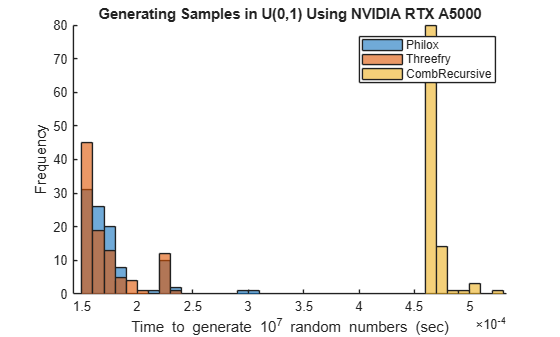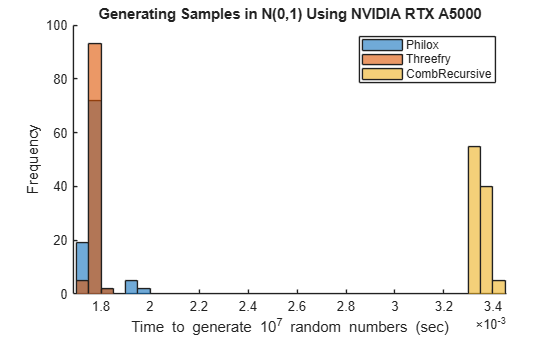Main Content

# Generating Random Numbers on a GPU

This example shows how to switch between the different random number generators that are supported on the GPU.

Random numbers form a key part of many simulation or estimation algorithms. Typically, these numbers are generated using the functions `rand`, `randi`, and `randn`. Parallel Computing Toolbox™ provides three corresponding functions for generating random numbers directly on a GPU: `rand`, `randi`, and `randn`. These functions can use one of several different number generation algorithms.

```d = gpuDevice; fprintf("This example is run on a " + d.Name + " GPU.")```
```This example is run on a GeForce GTX 1080 GPU. ```

### Discovering the GPU random number generators

The function `parallel.gpu.RandStream.list` provides a short description of the available generators.

`parallel.gpu.RandStream.list`
``` The following random number generator algorithms are available: MRG32K3A: Combined multiple recursive generator (supports parallel streams) Philox4x32_10: Philox 4x32 generator with 10 rounds (supports parallel streams) Threefry4x64_20: Threefry 4x64 generator with 20 rounds (supports parallel streams) ```

Each of these generators has been designed with parallel use in mind, providing multiple independent streams of random numbers. However, they each have some advantages and disadvantages:

• CombRecursive (also known as `MRG32k3a`): This generator was introduced in 1999 and has been widely tested and used.

• Philox (also known as Philox4x32_10): New generator introduced in 2011, specifically designed for high performance in highly parallel systems such as GPUs.

• Threefry (also known as Threefry4x64_20): New generator introduced in 2011 based on the existing cryptographic ThreeFish algorithm, which is widely tested and used. This generator was designed to give good performance in highly parallel systems such as GPUs. This is the default generator for GPU calculations.

The three generators available on the GPU are also available for use on the CPU in MATLAB®. The MATLAB generators have the same name and produce identical results given the same initial state. This is useful when you want to produce the same sets of random numbers on both the GPU and the CPU.

All of these generators pass the standard TestU01 test suite .

### Changing the default random number generator

The function `gpurng` can store and reset the generator state for the GPU. You can also use `gpurng` to switch between the different generators that are provided. Before changing the generator, store the existing state so that it can be restored at the end of these tests.

```oldState = gpurng; gpurng(0, "Philox4x32-10"); disp(gpurng)```
``` Type: 'philox' Seed: 0 State: [7×1 uint32] ```

### Generating uniformly distributed random numbers

Uniformly distributed random numbers are generated on the GPU using either `rand`, or `randi`. In performance terms, these two functions behave very similarly and only `rand` is measured here. `gputimeit` is used to measure the performance to ensure accurate timing results, automatically calling the function many times and correctly dealing with synchronization and other timing issues.

To compare the performance of the different generators, use `rand` to generate a large number of random numbers on the GPU using each generator. In the following code, `rand` generates $1{0}^{7}$ random numbers and is called 100 times for each generator. Each run is timed using `gputimeit`. Generating large samples of random numbers can take several minutes. The results indicate a performance comparison between the three random number generators available on the GPU.

```generators = ["Philox","Threefry","CombRecursive"]; gputimesU = nan(100,3); for g=1:numel(generators) % Set the generator gpurng(0, generators{g}); % Perform calculation 100 times, timing the generator for rep=1:100 gputimesU(rep,g) = gputimeit(@() rand(10000,1000,"gpuArray")); end end```
```% Plot the results figure hold on histogram(gputimesU(:,1),"BinWidth",1e-4); histogram(gputimesU(:,2),"BinWidth",1e-4); histogram(gputimesU(:,3),"BinWidth",1e-4) legend(generators) xlabel("Time to generate 10^7 random numbers (sec)") ylabel("Frequency") title("Generating samples in U(0,1) using " + d.Name) hold off```The newer generators Threefry and Philox have similar perfomance. Both are faster than CombRecursive.

### Generating normally distributed random numbers

Many simulations rely on perturbations sampled from a normal distribution. Similar to the uniform test, use `randn` to compare the performance of the three generators when generating normally distributed random numbers. Generating large samples of random numbers can take several minutes.

```generators = ["Philox","Threefry","CombRecursive"]; gputimesN = nan(100,3); for g=1:numel(generators) % Set the generator gpurng(0, generators{g}); % Perform calculation 100 times, timing the generator for rep=1:100 gputimesN(rep,g) = gputimeit(@() randn(10000,1000,"gpuArray")); end end```
```% Plot the results figure hold on histogram(gputimesN(:,1),"BinWidth",1e-4); histogram(gputimesN(:,2),"BinWidth",1e-4) histogram(gputimesN(:,3),"BinWidth",1e-4) legend(generators) xlabel("Time to generate 10^7 random numbers (sec)") ylabel("Frequency") title("Generating samples in N(0,1) using " + d.Name) hold off```Once again, the results indicate that the Threefry and Philox generators perform similarly and are both notably faster than CombRecursive. The extra work required to produce normally distributed values reduces the rate at which values are produced by each of the generators.

Before finishing, restore the original generator state.

`gpurng(oldState);`

### Conclusion

In this example, the three GPU random number generators are compared. The exact results vary depending on your GPU and computing platform. Each generator provides some advantages (`+`) and has some caveats (`-`).

Threefry

• (`+`) Fast

• (`+`) Based on well-known and well-tested Threefish algorithm

• (`-`) Relatively new in real-world usage

Philox

• (`+`) Fast

• (`-`) Relatively new in real-world usage

CombRecursive

• (`+`) Long track record in real-world usage

• (`-`) Slowest

### References

 L'Ecuyer, P., and R. Simard. "TestU01: A C library for empirical testing of random number generators." ACM Transactions on Mathematical Software. Vol. 33, No. 4, 2007, article 22.

## SupportGet trial now